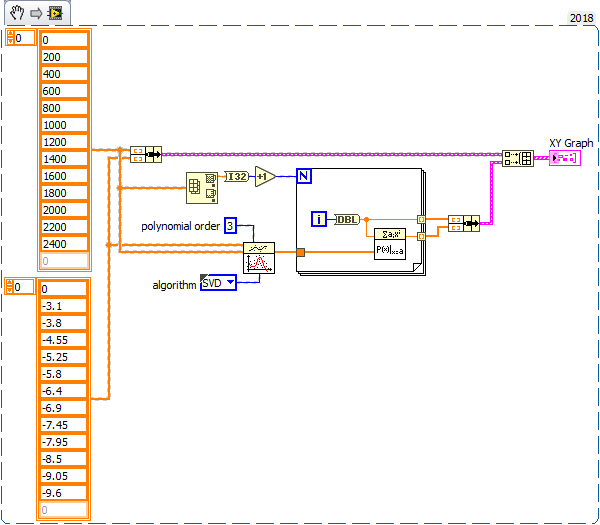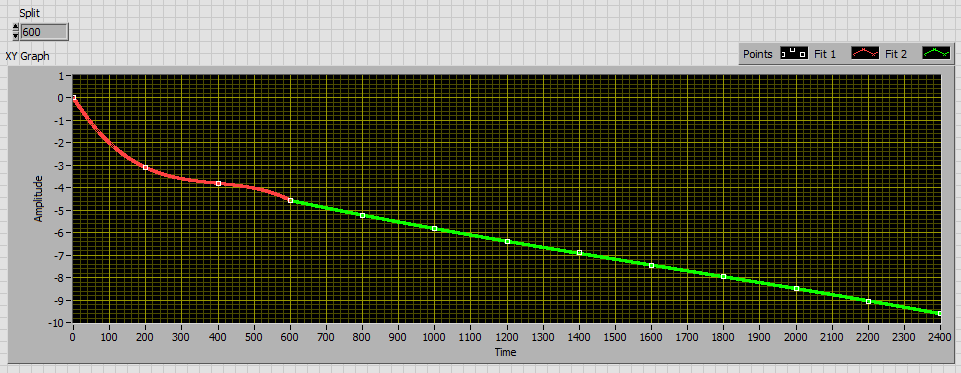# LabVIEW

cancel
Showing results for
Did you mean:

Solved!
Go to solution

## create a curve from points to get more values in labview 2021

Hi everyone,

I'm using labview 2021,

I need some help with a dashboard project I'm working on. I want to read sensors (let's say a torque sensor for a rotating shaft), and to get the final results correctly I have to consider the loss in the torque. So I made a trial free run for the system and I got the resistance torque for several rotational speeds. For example, I have n1=200, n2=400, n3=600 rpm, and so on, and for each speed I have a resistance torque, Q1, Q2, Q3, ...

My question is how to use these values to create a curve that can help me to get the resistance torque for any speed I want. For example, how can I make a curve from the above data and then use it to find Q for n=300, not 200 or 400.

I was thinking to use a fitting node to smooth the values I have first.

Thank you for your help. Any suggestion can be useful because I don't really know which direction I should go through.

Message 1 of 9
(564 Views)

## Re: create a curve from points to get more values in labview 2021

In order for us to be able to help you efficiently, please indicate the values you have measured and the expected accuracy of the correction.

A linear or polynomial interpolation seems to be the solution.

Message 2 of 9
(553 Views)

## Re: create a curve from points to get more values in labview 2021

Hi Ash-ta,

@Ash-ta wrote:

So I made a trial free run for the system and I got the resistance torque for several rotational speeds. For example, I have n1=200, n2=400, n3=600 rpm, and so on, and for each speed I have a resistance torque, Q1, Q2, Q3, ...

My question is how to use these values to create a curve that can help me to get the resistance torque for any speed I want. For example, how can I make a curve from the above data and then use it to find Q for n=300, not 200 or 400.

So you have a list of X-Y pairs and you want to get a polynomial fit for them?

What about using the polynomial fitting function (Math->Fitting->PolynomialFit)?

It will output the polynomial coefficients: use them with the PolynomialEvaluation function to calculate your "resistance torque" for each "rotational speed"…

Message 3 of 9
(551 Views)

## Re: create a curve from points to get more values in labview 2021

@JB please take a look at the attached image, it has the values of n and Q, although the values of Q look roughly like linear but I want to take the chance of writing here to learn the general case of nonlinear application,

@GerdW, do you mean like what I did in the attached Image?

I see the values are very far from the original table, for example, in the image I entered n= 0 and after the conversion it gave 1.188 which should be 0 based on the original tableMessage 4 of 9
(533 Views)
Solution
Accepted by topic author Ash-ta

## Re: create a curve from points to get more values in labview 2021

Example :If no interpolation method reduces the error sufficiently, I would separate the signal into several parts (two should suffice) to interpolate them separately.

Message 5 of 9
(529 Views)

## Re: create a curve from points to get more values in labview 2021

@JB wrote:

If no interpolation method reduces the error sufficiently, I would separate the signal into several parts (two should suffice) to interpolate them separately.

Your example answered my question thank you, however can you please tell me more what do you mean by " interpolation methods", do you have any suggestion about reducing the error?

We can notice from the curve that the resistance torque changes fast between 0 and 200 rpm, then it continues more like linear.

Changing the polynomial order helped, but still not enough.

I care more about speed between 0 to 1600 rpm, and i mostly care about getting 0 torque for 0 rpm because logically we can't have resistance torque unless the machine is turning.Message 6 of 9
(491 Views)
Solution
Accepted by topic author Ash-ta

## Re: create a curve from points to get more values in labview 2021

Hi Ash-ta,

play with all those options:Message 7 of 9
(481 Views)

## Re: create a curve from points to get more values in labview 2021

To refine the interpolation, it would also be necessary to have additional points between 0 and 200rpm.

Message 8 of 9
(463 Views)

## Re: create a curve from points to get more values in labview 2021

Here is an example of a polynomial fit by splitting the signal into two parts. As already mentioned, more points between 0 and 200 (400) rpm would be needed to get a better result in this area where the friction changes non-linearly.

It would then also be possible to lower the separation point of the signal and thus optimize the interpolation of its two parts.Message 9 of 9
(451 Views)